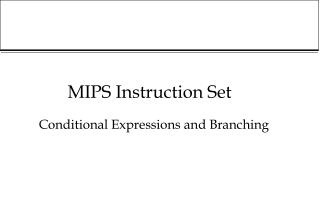DownloadDownload PresentationMIPS Instruction Set

# MIPS Instruction Set

Télécharger la présentation## MIPS Instruction Set

- - - - - - - - - - - - - - - - - - - - - - - - - - - E N D - - - - - - - - - - - - - - - - - - - - - - - - - - -
##### Presentation Transcript

1. MIPS Instruction Set Conditional Expressions and Branching

2. Outline • Motivation for conditional expressions and loops • How to add a sequence of numbers • Conditional expressions (branching) • Jumping • Switching

3. Last time ...adding 3 numbers... • What a mess this is... • lw \$10,A(\$0) # \$10 = a0 (\$0 always contains 0) • li \$4, 4 # \$4 = 4 • lw \$3,A(\$4) # \$3 = a1 • add \$10,\$10,\$3 # \$10 = a0 + a1 • li \$4, 8 # \$4 = 8 • lw \$3,A(\$4) # \$3 = a2 • add \$10,\$10,\$3 # \$10 = a0 + a1+ a2 • li \$4, 12 # \$4 = 12 • sw \$10,A(\$4) # a3 gets \$10 • Suppose 100 numbers to be added? • Doesn’t generalise!

4. Adding a sequence of n numbers • x, x, ..., x[n-1] sequence of n numbers.(n=100, say). • Consider (abstract representation) sum = 0 # initialise sum n = 100 # initialise boundary condition i = 0 # initialise counter LOOP: # label sum = sum + x[i] #overwrite sum i = i+1 #increment counter if (i != n) then go to LOOP

5. Adding the Sequence in MIPS move \$5,\$0 # puts 0 into \$5 (sum) addi \$4,\$0,100 # puts 100 into \$4 (n) move \$11,\$0 # puts 0 into \$11 (i) li \$12,4 # puts 4 into \$12 LOOP: mult \$14,\$11,\$12 # \$14 = i*4 (why?!) lw \$6,Xstart(\$14) # retrieves x[i] add \$5,\$5,\$6 # sum = sum + x[i] addi \$11,\$11,1 # i = i+1 bne \$11,\$4, LOOP # if i < n go to LOOP sw \$5,Sum(\$0) #result put in memory

6. Conditional Expressions • bne \$a,\$b,LABEL • branch if not equal • if \$a != \$b then jump to LABEL • beq \$a,\$b,LABEL • branch if equal • if \$a == \$b jumpt to LABEL • slt \$a,\$b,\$c • set if less than • if (\$b < \$c) then \$a = 1 else \$a = 0

7. Example (introduces jump) • Consider the following abstract code if (a < b) then c = 100 else c = 500 • In MIPS (suppose a,b,c are \$5,\$6,\$7) slt \$10,\$5,\$6 # \$10 = 1 if \$5<\$6 beq \$10,\$0,ELSE # if \$10==0 goto else addi \$7,\$0,100 # \$7 =100 j CONTINUE # jump to continue ELSE : addi \$7,\$0,500 # \$7 = 500 CONTINUE: whatever here...

8. Choosing from several alternatives • Abstract code if k=0 then a=20 else if k=1 then a=10 else if k=2 then a=11; • C/C++ representation (uses ‘switch’) switch(k){ 0 : a = 20; break; 1 : a = 10; break; 2 : a = 11; }

9. Using jump register (jr \$a) • jumps to instruction referenced in \$a • suppose Label is memory location containing addresses L0, L1, L2. (\$4=k*4) lw \$10,Label(\$4) # \$10 = Label[k] jr \$10 # jump \$10 L0: addi \$20,\$0,20 # \$20 gets 20 j BREAK L1: addi \$20,\$0,10 # \$20 gets 10 j BREAK L2: addi \$20,\$0,11 # \$20 gets 11 BREAK: continue whatever here

10. Summary • bne \$a,\$b,LABEL #branch if not equal • beq \$a,\$b,LABEL #branch if equal • slt \$a,\$b,\$c # select if less than • j LABEL #jump to the instruction referenced by LABEL • jr \$a #jump to instruction referenced in register \$a

11. Still potential for a mess!... • Adding 100 numbers x[] is fine. • Suppose later we want to add 1011 numbers using sequence y[]? • Do we have to repeat the same set of instructions all over again, with small modifications? • No way! • Use procedures!!!